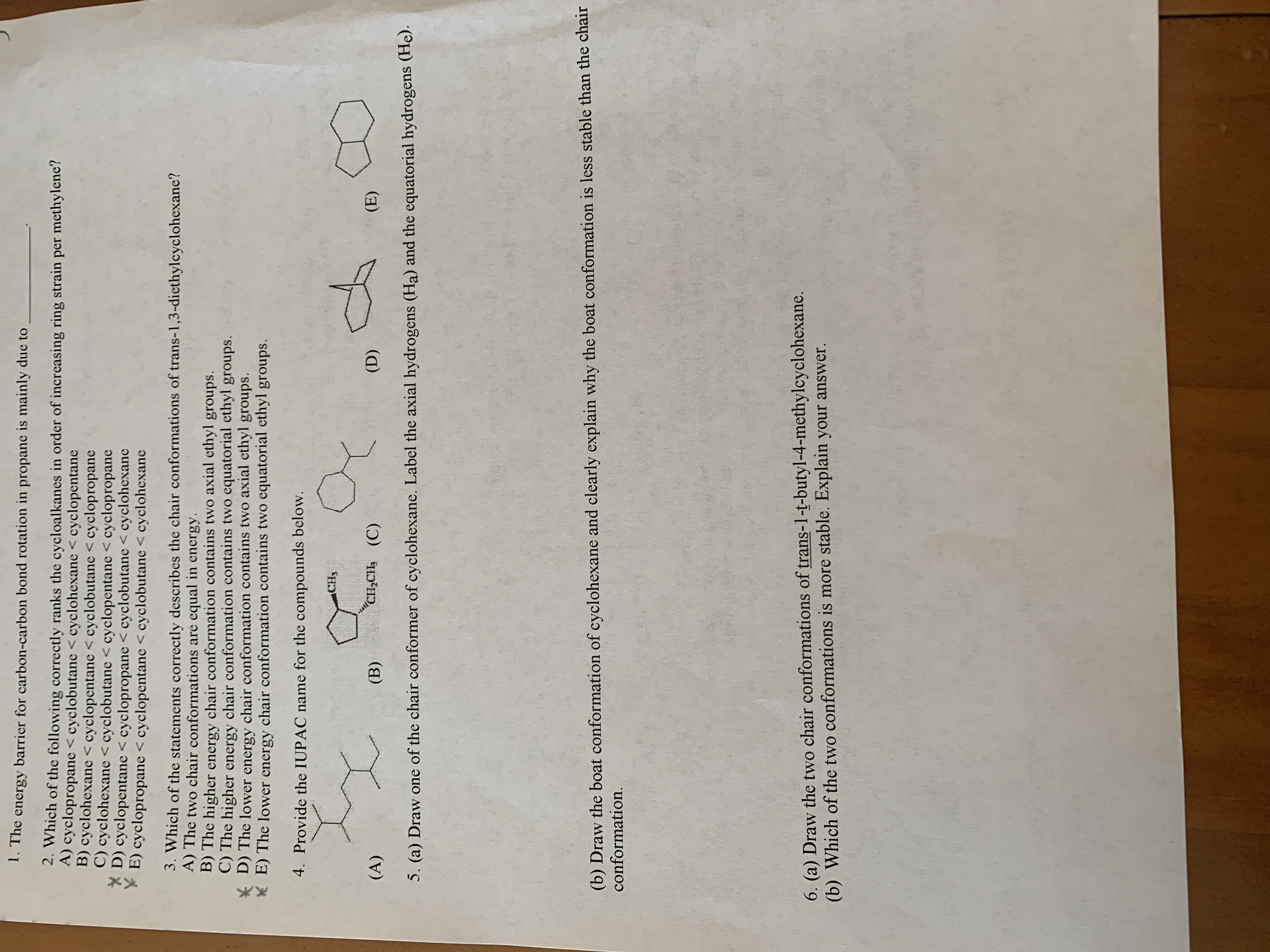# 1. The energy barrier for carbon-carbon bond rotation in propane is mainly due to2. Which of the following correctly ranks the cycloalkanes in order of increasing ring strain per methylene'?A) cyclopropane < cyclobutane < cyclohexane < cyclopentaneB) cyclohexane < cyclopentane < cyclobutane < cyclopropaneC) cyclohexane < cyclobutane < cyclopentane < cyclopropaneD) cyclopentane < cyclopropane < cyclobutane < cyclohexaneE) cyclopropane < cyclopentane < cyclobutane < cyclohexane3. Which of the statements correctly describes the chair conformations of trans-1,3-diethylcyclohexane?A) The two chair conformations are equal in energy.B) The higher energy chair conformation contains two axial ethyl groups.C) The higher energy chair conformation contains two equatorial ethyl groups.D) The lowerE) The lower energy chair conformation contains two equatorial ethyl groups.chair conformation contains two axial ethyl groups.energy4. Provide the IUPAC name for the compounds below.CH3"С,CHa (C)(E)(D)(A)(В)5. (a) Draw one of the chair conformer of cyclohexane. Label the axial hydrogens (Ha) and the equatorial hydrogens (He).(b) Draw the boat conformation of cyclohexane and clearly explain why the boat conformation is less stable than the chairconformation.6. (a) Draw the two chair conformations of trans-1-t-butyl-4-methylcyclohexane.(b) Which of the two conformations is more stable. Explain your answer.

Question
128 viewshelp_outlineImage Transcriptionclose1. The energy barrier for carbon-carbon bond rotation in propane is mainly due to 2. Which of the following correctly ranks the cycloalkanes in order of increasing ring strain per methylene'? A) cyclopropane < cyclobutane < cyclohexane < cyclopentane B) cyclohexane < cyclopentane < cyclobutane < cyclopropane C) cyclohexane < cyclobutane < cyclopentane < cyclopropane D) cyclopentane < cyclopropane < cyclobutane < cyclohexane E) cyclopropane < cyclopentane < cyclobutane < cyclohexane 3. Which of the statements correctly describes the chair conformations of trans-1,3-diethylcyclohexane? A) The two chair conformations are equal in energy. B) The higher energy chair conformation contains two axial ethyl groups. C) The higher energy chair conformation contains two equatorial ethyl groups. D) The lower E) The lower energy chair conformation contains two equatorial ethyl groups. chair conformation contains two axial ethyl groups. energy 4. Provide the IUPAC name for the compounds below. CH3 "С,CHa (C) (E) (D) (A) (В) 5. (a) Draw one of the chair conformer of cyclohexane. Label the axial hydrogens (Ha) and the equatorial hydrogens (He). (b) Draw the boat conformation of cyclohexane and clearly explain why the boat conformation is less stable than the chair conformation. 6. (a) Draw the two chair conformations of trans-1-t-butyl-4-methylcyclohexane. (b) Which of the two conformations is more stable. Explain your answer. fullscreen
check_circle

Step 1

Propane is represented in Newman's pro...

### Want to see the full answer?

See Solution

#### Want to see this answer and more?

Solutions are written by subject experts who are available 24/7. Questions are typically answered within 1 hour.*

See Solution
*Response times may vary by subject and question.
Tagged in

### Chemistry